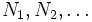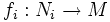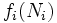# Manifold is not union of images of manifolds of smaller dimension

This fact is an application of the following pivotal fact/result/idea: Sard's theorem
View other applications of Sard's theorem OR Read a survey article on applying Sard's theorem

This article gives a purely topological (or set-theoretic) constraint that we get when we restrict ourselves to the category of differential manifolds with smooth maps

## Statement

Let$M$ be a smooth manifold and$N_1, N_2, \ldots$ be a countable sequence of smooth manifolds, each having dimension strictly less than that of$M$. Suppose$f_i:N_i \to M$ are smooth maps. Then,$M$ is not the union of$f_i(N_i)$.

The analogous statement is not true for topological manifolds with continuous maps: there can be a continuous surjective map from a manifold of smaller dimension to a manifold of larger dimension.